# CBSE Class 9 Maths Chapter 5 Introduction to Euclids Geometry

Download CBSE Class 9 Maths Chapter 5 Introduction To Euclids Geometry In Pdf

Chapter 5 CBSE Class 9 Maths Introduction to Euclid's Geometry

## CBSE Class 9 Maths Chapter 5 Introduction to Euclid's Geometry

The Greeks developed geometry is a systematic manner Euclid (300 B.C.) a greek mathematician, father of geometry introduced the method of proving mathematical results by using deductive logical reasoning and the previously proved result. The Geometry of plane figure is know as "Euclidean Geometry". Axioms :

The basic facts which are taken for granted without proof are called axioms some Euclid's axioms are
(i) Things which are equal to the same thing are equal to one another. i.e.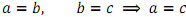i) If equals are added to equals, the wholes are equal i.e.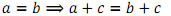(iii) If equals are subtracted from equals, the remainders are equal i.e.
(iv) Things which coincide with one another are equal to one another. (v) The whole is greater than the part.

### Postulates :

Axioms are the general statements, postulates are the axioms relating to a particular field.
Educlid's five postulates are.
(i) A straight line may be drawn from any one point to any other point.
(ii) A terminated line can be produced indefinitely.
(iii) A circle can be drawn with any centre and any radius.
(iv) All right angles are equal to one another.
(v) If a straight line falling on two straight lines makes the interior angles on the same side of it taken together less than two right angles, then the two straight lines, if produced indefinitely meet on that side on which the angles are less than two right angles.

Statements : A sentence which is either true or false but not both, is called a statement.
eg. (i) 4+9=6 If is a false sentence, so it is a statement.
(ii) Sajnay is tall. This is not a statement because he may be tall for certain persons and may not be taller for others.

Theorems : A statement that requires a proof is called a theorem.
eg. (i) The sum of the angles of triangle is 180o
.    (ii) The angles opposite to equal sides of a triangles are equal.
Corollary - Result deduced from a theorem is called its corollary.

### Section - A

1) Euclid stated that if equals are subtracted from equals, the remainders are equals in the forms of
(a) an axiom
(b) a postulate
(c) a definition
(d) a proof
Ans.(a)

(2) Euclid stated that all right angles are equals to each other in the form of
(a) an axiom
(b) a definition
(c) a postulate(
d) a proof
Ans.(c)

(3) Which of the following needs a proof:
(a) Theorem
(b) Axiom
(c) Definition
(d) Postulate Ans.(a)

(4) The number of dimensions, a solid has
(a) 1
(b) 2
(c) 3
(d) 0
Ans.(c)

(5) The number of dimensions a surface has :
(a) 1
(b) 2
(c) 3
(d) 0
Ans.(b)

(6) The number of dimensions, a point has
(a) 0
(b) 1
(c) 2
(d) 3
Ans.(a)

(7) Which one of the following statement is true?
(a) Only one line can pass through a single point.
(b) There are infinite number of lines which pass through two distinct points.
(c) Two distinct lines cannot have more than one point in common. (d) If two circles are equal, then their radii are not equal.
Ans.(c)

(8) Euclid divided his famous treatise "The Element" into
(a) 13 chapters
(b) 12 Chapters
(c) 11 Chapters
(d) 9 Chapters
Ans.(a)

(9) Thales belongs to the country.
(a) Babylonia
(b) Egypt
(c) Greece
(d) Rome
Ans.(c)

### Section - B

(10) If AB=CD can you say that AC=BD?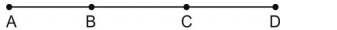(11) In how many points two distinct lines can intersect.

(12) In how many lines two distinct planes can intersect.

### Section - C

(13) If a point C lies between two points A and B such that AC=CB then prove that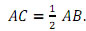Explain by drawing the figure.

(14) In the figure, AC=BD prove that AB=CD.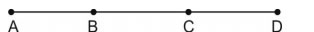(15) If C is called a mid point of line segment AB. Prove that every line segment has one and only one mid point.

(16) Define the following terms :
(i) Parallel lines
(ii) Concurrent lines
(iii) Intersecting lines

(17) State Euclid's any three postulates.

(18) State Euclid's any three axioms.### NCERT Books Free Pdf Download for Class 5, 6, 7, 8, 9, 10 , 11, 12 Hindi and English Medium

 Mathematics Biology Psychology Chemistry English Economics Sociology Hindi Business Studies Geography Science Political Science Statistics Physics Accountancy